# Indexes

NTFS uses index data structures in many situations, and this section describes them. An index in NTFS is a collection of attributes that is stored in a sorted order. The most common usage of an index is in a directory because directories contain \$FILE_NAME attributes.

Prior to version 3.0 of NTFS (which came with Windows 2000), only the \$FILE_NAME attribute was in an index, but now there are several other uses of indexes and they contain different attributes. For example, security information is stored in an index, as is quota information. This section shows what an index looks like and how it is implemented.

B-Trees

An NTFS index sorts attributes into a tree, specifically a B-tree. A tree is a group of data structures called nodes that are linked together such that there is a head node and it branches out to the other nodes. Consider Figure 11.13(A), where we see node A on top and it links to nodes B and C. Node B links to node D and E. A parent node is one that links to other nodes, and a child node is one that is linked to. For example, A is a parent node to B and C, which are children of A. A leaf node is one that has no links from it. Nodes C, D, and E are leaves. The example shown is a binary tree because there are a maximum of two children per node.

Figure 11.13. Examples of A) a tree with 5 nodes and B) the same tree that is sorted by the node values.Trees are useful because they can be used to easily sort and find data. Figure 11.13(B) shows the same tree as we saw on the left side, but now with values that are assigned to each node. If we are trying to look up a value, we compare it to the root node. If the root node is larger, we go to the child on the left. If the root node is smaller, we go to the child on the right. For example, if we want to find the value 6, we compare it to the root value, which is 7. The node is larger, so we go to the left-hand child and compare its value, which is 5. This node is smaller, so we go to the right-hand child and compare its value, which is 6. We have found our value with only three comparisons. We could have found the value 9 in only two comparisons instead of five if the values were in a list.

NTFS uses B-trees, which are similar to the binary tree we just saw, but there can be more than two children per node. Typically, the number of children that a node has is based on how many values each node can store. For example, in the binary tree we stored one value in each node and had two children. If we can store five values in each node, we can have six children. There are many variations of B-trees, and there are more rules than I will describe here because the purpose of this section is to describe their concepts, not to describe how you can create a B-tree.

Figure 11.14 shows a B-tree with names as values instead of numbers. Node A contains three values and four children. If we were looking for the file ggg.txt, we would look at the values in the root node and determine that the name alphabetically falls in between eee.txt and lll.txt. Therefore, we process node C and look at its values. We find the file name in that node.

Figure 11.14. A B-tree with file names as values.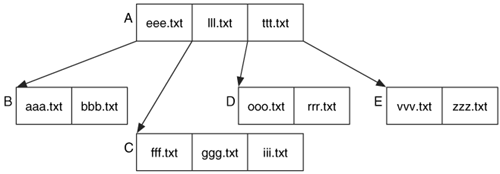Now let's make this complex by looking at how values are added and deleted. This is an important concept because it explains why deleted file names are difficult to find in NTFS. Let us assume that we can fit only three file names per node, and the file jjj.txt is added. That sounds easy, but as we will see it results in two nodes being deleted and five new nodes being created. When we locate where jjj.txt should fit, we identify that it should be at the end of node C, following the iii.txt name. The top of Figure 11.15 shows this situation, but unfortunately, there are now four names in this node, and it can fit only three. Therefore, we break node C in half, move ggg.txt up a level, and create nodes F and G with the resulting names from node C. This is shown at the bottom of Figure 11.15.

Figure 11.15. The top tree shows 'jjj.txt' added to node C, and the bottom tree is the result of removing node C because each node can have only three names.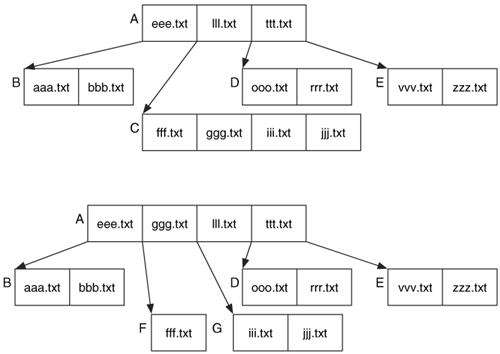Unfortunately, node A now has four values in it. So we divide it in half and move ggg.txt to the top-most node. The final result can be found in Figure 11.16. Adding one file results in removing nodes A and C and adding nodes F, G, H, I, and J. Any remnant data in nodes A and C from previously deleted files may now be gone.

Figure 11.16. The final state from adding the 'jjj.txt' file.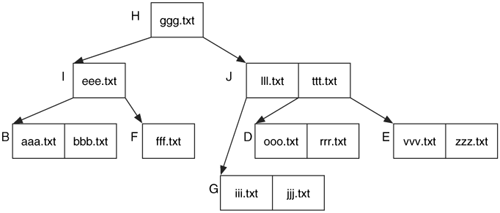Now delete the zzz.txt file. This action removes the name from node E and does not require any other changes. Depending on the implementation, the details of zzz.txt file may still exist in the node and could be recovered.

To make things more difficult, consider if fff.txt was deleted. Node F becomes empty, and we need to fill it in. We move eee.txt from node I to node F and move bbb.txt from node B to node I. This creates a tree that is still balanced where all leaves are the same distance from node H. The resulting state is found in Figure 11.17.

Figure 11.17. Tree after deleting the 'zzz.txt' file and the 'fff.txt' file.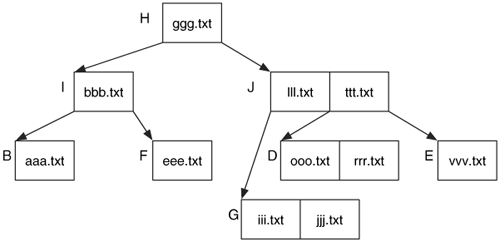Node B will contain bbb.txt in its unallocated space because bbb.txt was moved to node I. Our analysis tool might show that the bbb.txt file was deleted, but it really wasn't. It was simply moved because fff.txt was deleted.

The point of walking through the process of adding and deleting values from the tree was to show how complex the process could be. With file systems, such as FAT, that use a list of names in a directory, it is easy to describe why a deleted name does or does not exist, but with trees it is very difficult to predict the end result.

NTFS Index Attributes

Now that we have described the general concept of B-trees, we need to describe how they are implemented in NTFS to create indexes. Each entry in the tree uses a data structure called an index entry to store the values in each node. There are many types of index entries, but they all have the same standard header fields, which are given in Chapter 13. For example, a directory index entry contains a few header values and a \$FILE_NAME attribute. The index entries are organized into nodes of the tree and stored in a list. An empty entry is used to signal the end of the list. Figure 11.18 shows an example of a node in a directory index with four \$FILE_NAME index entries.

Figure 11.18. A node in an NTFS directory index tree with four index entries.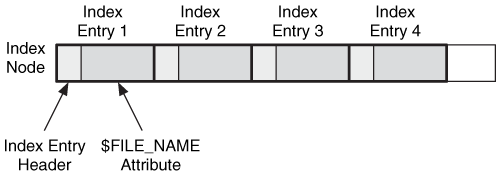The index nodes can be stored in two types of MFT entry attributes. The \$INDEX_ROOT attribute is always resident and can store only one node that contains a small number of index entries. The \$INDEX_ROOT attribute is always the root of the index tree.

Larger indexes allocate a non-resident \$INDEX_ALLOCATION attribute, which can contain as many nodes as needed. The content of this attribute is a large buffer that contains one or more index records. An index record has a static size, typically 4,096 bytes, and it contains a list of index entries. Each index record is given an address, starting with 0. We can see this in Figure 11.19 where we have an \$INDEX_ROOT attribute with three index entries and a non-resident \$INDEX_ALLOCATION attribute that has allocated cluster 713, and it uses three index records.

Figure 11.19. This directory has three index entries in its resident \$INDEX_ROOT attribute and three index records in its non-resident \$INDEX_ALLOCATION attribute.An \$INDEX_ALLOCATION attribute can have allocated space that is not being used for index records. The \$BITMAP attribute is used to manage the allocation status of the index records. If a new node needs to be allocated for the tree, \$BITMAP is used to find an available index record; otherwise, more space is added. Each index is given a name and the \$INDEX_ROOT, \$INDEX_ALLOCATION, and \$BITMAP attributes for the index are all assigned the same name in their attribute header.

Each index entry has a flag that shows if it has any children nodes. If there are children nodes, their index record addresses are given in the index entry. The index entries in a node are in a sorted order, and if the value you are looking for is smaller than the index entry and the index entry has a child, you look at its child. If you get to the empty entry at the end of the list, you look at its child.

Let us go over some examples. Consider an index with three entries that fit into \$INDEX_ROOT. In this case, only a \$INDEX_ROOT is allocated, and it contains three index entry data structures and the empty entry at the end of the list. This can be seen in Figure 11.20(A). Now consider an index with 15 entries, which do not fit into an \$INDEX_ROOT but fit into an \$INDEX_ALLOCATION attribute with one index record. It can be seen in Figure 11.20(B). After we fill up the index entries in the index record, we need to add a new level, and we create a three-node tree. This scenario is shown in Figure 11.20(C). This has one value in the root node and two children nodes. Each child node is located in a separate index record in the same \$INDEX_ALLOCATION attribute, and it is pointed to by the entries in the \$INDEX_ROOT node.

Figure 11.20. Three scenarios of NTFS indexes, including A) a small index of three entries, B) a larger index with two nodes and 15 entries, and C) a three-node tree with 25 entries.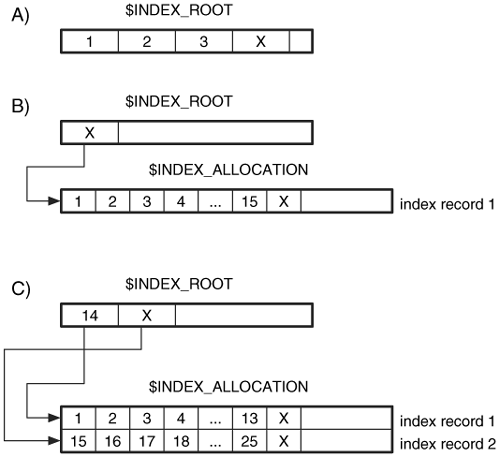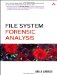File System Forensic Analysis
ISBN: 0321268172
EAN: 2147483647
Year: 2006
Pages: 184
Authors: Brian Carrier